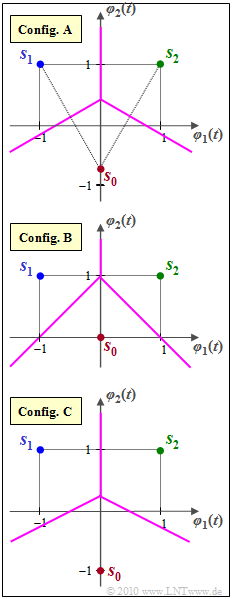# Exercise 4.10: Union BoundSignal space constellations with $N=2$  and  $M=3$

The so-called  "Union Bound"  gives an upper bound for the error probability of a non-binary transmission system  $(M > 2)$.

• The actual  (average)  error probability is generally given as follows:
$${\rm Pr}({ \cal E}) \hspace{-0.1cm} \ = \ \hspace{-0.1cm} \sum\limits_{i = 0 }^{M-1} {\rm Pr}(m_i) \cdot {\rm Pr}({ \cal E}\hspace{0.05cm}|\hspace{0.05cm} m_i ) \hspace{0.05cm},$$
$${\rm Pr}({ \cal E}\hspace{0.05cm}|\hspace{0.05cm} m_i ) \hspace{-0.1cm} \ = \ \hspace{-0.1cm} {\rm Pr} \left [ \bigcup_{k \ne i} { \cal E}_{ik}\right ] \hspace{0.05cm},\hspace{0.2cm}{ \rm where}\hspace{0.2cm} { \cal E}_{ik}\text{:} \ \ \boldsymbol{ r }{\rm \hspace{0.15cm}is \hspace{0.15cm}closer \hspace{0.15cm}to \hspace{0.15cm}}\boldsymbol{ s }_k {\rm \hspace{0.15cm}than \hspace{0.15cm}to \hspace{0.15cm}the \hspace{0.15cm}setpoint \hspace{0.15cm}}\boldsymbol{ s }_i \hspace{0.05cm}.$$
• The simpler  "Union Bound"  $\rm (UB)$  provides an upper bound on the falsification probability assuming that message  $m_i$  $($or signal  $\boldsymbol{s}_i)$  was sent:
$$p_{{\rm UB}\hspace{0.05cm}| \hspace{0.05cm}\boldsymbol{ s }_i} \hspace{-0.1cm} \ \ge \ \hspace{-0.1cm} {\rm Pr}({ \cal E}\hspace{0.05cm}|\hspace{0.05cm} \boldsymbol{ s }_i ) = {\rm Pr}({ \cal E}\hspace{0.05cm}|\hspace{0.05cm} m_i )\hspace{0.05cm},\$$
$$p_{{\rm UB}\hspace{0.05cm}| \hspace{0.05cm}\boldsymbol{ s }_i} \hspace{-0.1cm} \ = \ \hspace{-0.2cm}\sum\limits_{k = 0 ,\hspace{0.1cm} k \ne i}^{M-1}\hspace{-0.1cm} {\rm Pr}({ \cal E}_{ik}) = \hspace{-0.1cm}\sum\limits_{k = 0, \hspace{0.1cm} k \ne i}^{M-1}\hspace{-0.1cm}{\rm Q} \left ( \frac{d_{ik}/2}{\sigma_n} \right )\hspace{0.05cm}.$$

The following abbreviations are used:

• ${\rm Q}(x)$  is the complementary Gaussian error function;
• $d_{ik}$  denotes the distance between the signal points  $\boldsymbol{s}_i$  and $\boldsymbol{s}_k$;
• $\sigma_n$  is the rms value  (⇒ root of the variance)  of the additive white Gaussian noise.

⇒   By averaging over all possible signals  $\boldsymbol{s}_i$,  we then arrive at the actual  "Union Bound":

$$p_{\rm UB} = \sum\limits_{i = 0 }^{M-1} {\rm Pr}(\boldsymbol{ s }_i) \cdot p_{{\rm UB}\hspace{0.05cm}| \hspace{0.05cm}\boldsymbol{ s }_i} \ge {\rm Pr}({ \cal E}) \hspace{0.05cm}.$$
• The graph shows three different signal space constellations,
each with  $M = 3$  signal space points  $\boldsymbol{s}_0$,  $\boldsymbol{s}_1$  and  $\boldsymbol{s}_2$  in two-dimensional space  $(N = 2)$.
• The basis functions  $\varphi_1(t)$  and  $\varphi_2(t)$  are suitably normalized.
• Thus,  the signal space coordinates are also pure numerical values without unit:
$$\boldsymbol{ s }_1 = (-1, \hspace{0.1cm}+1)\hspace{0.05cm}, \hspace{0.2cm} \boldsymbol{ s }_2 = (+1, \hspace{0.1cm}+1)\hspace{0.05cm}.$$
• The signal space point  $\boldsymbol{s}_0$  in configuration  $\rm A$  is located such that  $\boldsymbol{s}_0$,  $\boldsymbol{s}_1$,  $\boldsymbol{s}_2$  describe an equilateral triangle.
• In contrast,  in configurations  $\rm B$  and  $\rm C$,    $\boldsymbol{s}_0 = (0,\ 0)$  resp.  $\boldsymbol{s}_0 = (0, \ –1)$.

Notes:

• Use the AWGN rms value  $\sigma_n = 0.5$  for all calculations.
• Given the following values of the complementary Gaussian error function:
$${\rm Q}(1) \hspace{-0.1cm} \ \approx \ \hspace{-0.1cm} 0.159\hspace{0.05cm}, \hspace{0.2cm}{\rm Q}(\sqrt{2}) \approx 0.079\hspace{0.05cm}, \hspace{0.23cm}{\rm Q}(\sqrt{3}) \approx 0.042\hspace{0.05cm},$$
$${\rm Q}(2) \hspace{-0.1cm} \ \approx \ \hspace{-0.1cm} 0.023\hspace{0.05cm}, \hspace{0.2cm}{\rm Q}(2.14) \approx 0.016\hspace{0.05cm}, \hspace{0.1cm}{\rm Q}(\sqrt{5}) \approx 0.013 \hspace{0.05cm}.$$

### Questions

1

Which of the three configurations leads to the smallest error probability  $($at least according to the Union Bound approximation$)$?

 Configuration  $\rm A$, Configuration  $\rm B$, Configuration  $\rm C$.

2

Calculate the  "averaged Union Bound"  $(p_{\rm UB})$  for configuration  $\rm A$.

 $p_{\rm UB} \ = \$ $\ \%$

3

Calculate the  "averaged Union Bound"  for configuration  $\rm B$.

 $p_{\rm UB} \ = \$ $\ \%$

4

Calculate the  "averaged Union Bound"  for configuration  $\rm C$.

 $p_{\rm UB} \ = \$ $\ \%$

5

How would the noise rms value  $\sigma_n$  have to be changed for configuration  $\rm A$  to yield the same  "Union Bound"  as in subtask  (4)?

 $\sigma_n \ = \$

### Solution

#### Solution

(1)  Points  $\boldsymbol{s}_1$  and  $\boldsymbol{s}_2$  are the same for all configurations.

• The smallest error probability occurs when  $\boldsymbol{s}_0$  is farthest from  $\boldsymbol{s}_1$  and  $\boldsymbol{s}_2$.
• This is the case for configuration  $\rm C$   ⇒   solution 3.

(2)  For configuration  $\rm A$,  the distance between all points is the same:  $d_{01} = d_{02} = d_{12} = 2$.

• Therefore,  to calculate the  "Union Bound",  it is not necessary to average over all symbols.
• And it is valid since e.g.  $\boldsymbol{s}_0$  is distorted into symbol  $\boldsymbol{s}_1$  or  $\boldsymbol{s}_2$  with equal probability:
$${\rm Pr}({ \cal E}) \le p_{\rm UB} = 2 \cdot {\rm Q} \left ( \frac{d_{ik}/2}{\sigma_n} \right ) = 2 \cdot {\rm Q}(2) \approx 2 \cdot 0.023 \hspace{0.1cm}\hspace{0.15cm}\underline {= 4.6\%} \hspace{0.05cm}.$$

(3)  Here,  the falsification probabilities differ for each symbol.

• If  $\boldsymbol{s}_0$  was sent,  with $d_{01} = d_{02} = 2^{0.5}$  and  $\sigma = 0.5$:
$$p_{{\rm UB}\hspace{0.05cm}| \hspace{0.05cm}\boldsymbol{ s }_0} = 2 \cdot {\rm Q} \left ( \frac{\sqrt{2}/2}{0.5} \right ) = 2 \cdot {\rm Q}(\sqrt{2}) = 2 \cdot 0.079 = 0.158 \hspace{0.05cm}.$$
• In contrast,  the other two conditional probabilities are smaller:
$$p_{{\rm UB}\hspace{0.05cm}| \hspace{0.05cm}\boldsymbol{ s }_1} = p_{{\rm UB}\hspace{0.05cm}| \hspace{0.05cm}\boldsymbol{ s }_2} \hspace{-0.1cm} \ = \ \hspace{-0.1cm} {\rm Q} \left ( \frac{{2}/2}{0.5} \right )+{\rm Q} \left ( \frac{\sqrt{2}/2}{0.5} \right )= {\rm Q}(2) +{\rm Q}(\sqrt{2}) = 0.023 + 0.079 = 0.102 \hspace{0.05cm}.$$
• By averaging,  we obtain for the Union Bound considering the different distances:
$$p_{\rm UB} \hspace{-0.1cm} \ = \ \hspace{-0.1cm} {1}/{3} \cdot \left [ p_{{\rm UB}\hspace{0.05cm}| \hspace{0.05cm}\boldsymbol{ s }_0} + p_{{\rm UB}\hspace{0.05cm}| \hspace{0.05cm}\boldsymbol{ s }_1} +p_{{\rm UB}\hspace{0.05cm}| \hspace{0.05cm}\boldsymbol{ s }_2}\right ]= {1}/{3} \cdot \left [ 2 \cdot {\rm Q}(\sqrt{2})+ 2 \cdot ({\rm Q}({2}) + {\rm Q}(\sqrt{2})) \right ] = {1}/{3} \cdot \left [ 4 \cdot {\rm Q}(\sqrt{2})+ 2 \cdot {\rm Q}({2}) \right ]$$
$$\Rightarrow \hspace{0.3cm} p_{\rm UB} = {1}/{3} \cdot \big [ 4 \cdot 0.079+ 2 \cdot 0.023 \big ] \hspace{0.1cm}\hspace{0.12cm}\underline {\approx 12.1\% } \hspace{0.3cm}\Rightarrow \hspace{0.3cm} p_{\rm UB}\ge {\rm Pr}({ \cal E})\hspace{0.05cm}.$$

(4)  This configuration is described by the following equations:

$$d_{01} = d_{02} = \sqrt{2^2 + 1^2}= \sqrt{5} \approx 2.24\hspace{0.2cm}, d_{12} = 2$$
$$\Rightarrow \hspace{0.3cm} p_{\rm UB} = {1}/{3} \cdot \big [ 4 \cdot {\rm Q}(\sqrt{5})+ 2 \cdot {\rm Q}({2}) \big ] = {1}/{3} \cdot \big [ 4 \cdot 0.013+ 2 \cdot 0.023 \big ]\hspace{0.1cm}\hspace{0.15cm}\underline {\approx 3.2\%} \hspace{0.05cm}.$$

(5)  Let it be:

$$p_{\rm UB} = 2 \cdot {\rm Q}\left ( {1}/{\sigma_n} \right ) = 0.032 \hspace{0.3cm} \Rightarrow \hspace{0.3cm} {\rm Q}\left ( {1}/{\sigma_n} \right ) = 0.016\hspace{0.3cm} \Rightarrow \hspace{0.3cm} {1}/{\sigma_n} \approx 2.14 \hspace{0.3cm} \Rightarrow \hspace{0.3cm}\hspace{0.1cm}\hspace{0.15cm}\sigma_n \hspace{0.15cm}\underline {\approx 0.467}\hspace{0.05cm}.$$